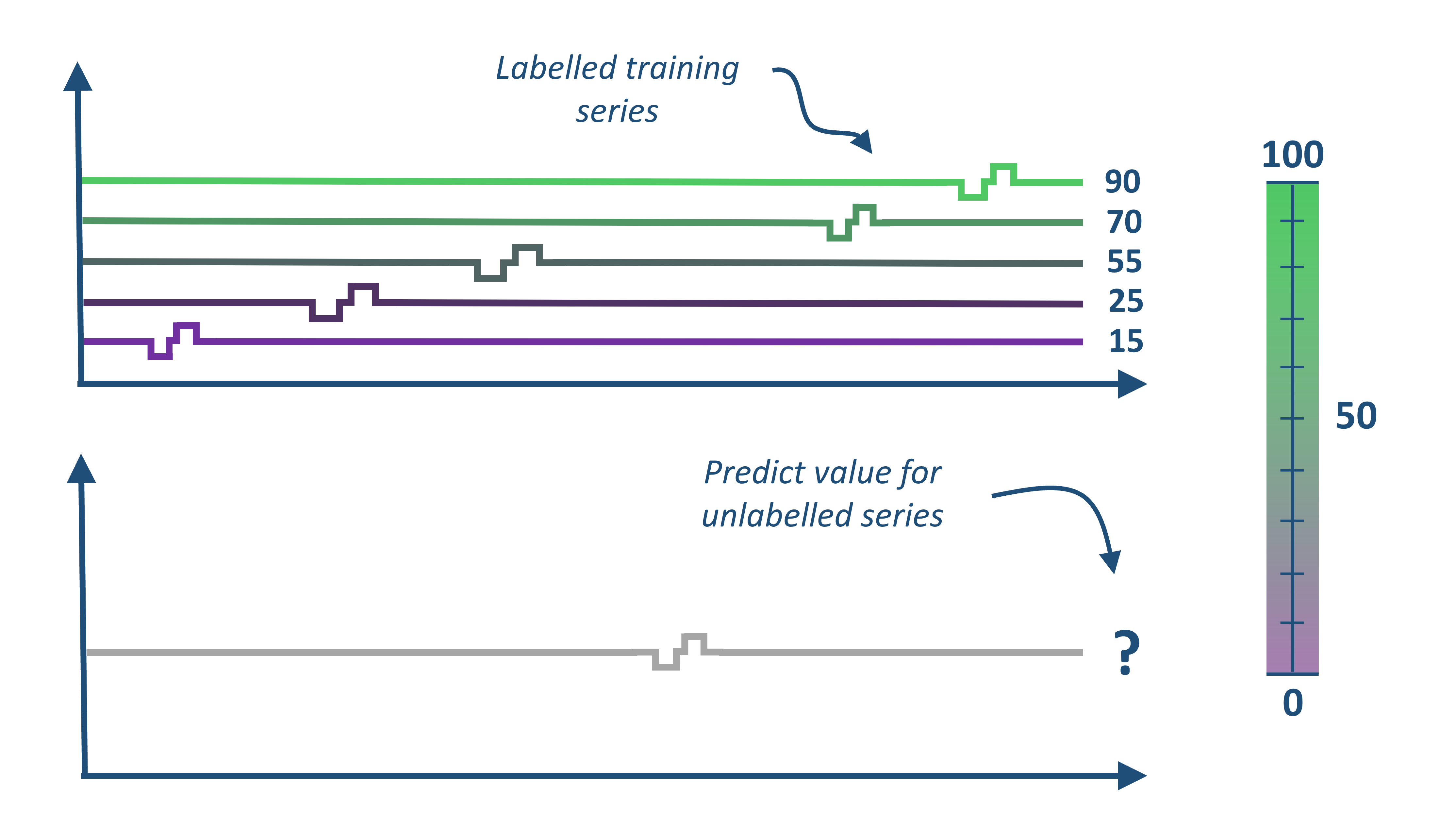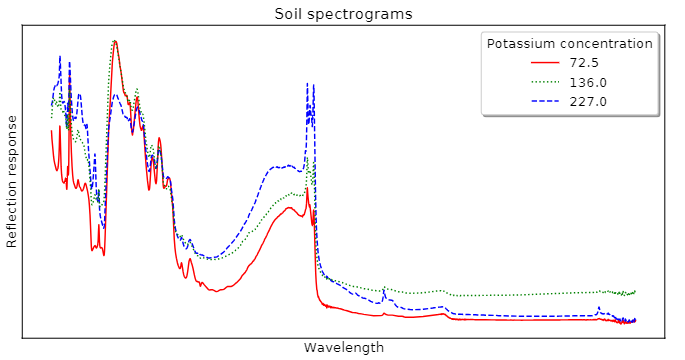# Time Series Extrinsic Regression (TSER) with aeon#

Time Series Regression (TSR) involves training a model from a collection of time series (real valued, ordered, data) in order to predict a continuous target variable.

There are two types of TSR.

1. Time Series Forecasting Regression (TSFR) relates to forecasting reduced to regression through a sliding window. This is the more familiar type to most people. The notebook for forecasting using supervised regressors can be found here.

2. Time Series Extrinsic Regression (TSER) was formally specified in Tan et al.  as a related, but distinct, type of time series regression problem. Rather than being derived from a forecasting problem, TSER involves a predictive model built on time series to predict a real-valued variable distinct from the training input series.

This notebook focuses on the aeon regression module, which consists of algorithms for TSER.An example TSER problem is shown in the below image of soil spectrograms which can be used to estimate the potassium concentration. Ground truth is found through expensive lab based experiments that take some time. Spectrograms (ordered data series we treat as time series) are cheap to obtain and the data can be collected in any environment. An accurate regressor from spectrogram to concentration would make land and crop management more efficient.## Data Storage and Problem Types#

Regressors take time series input as either 3D numpy of shape(n_cases, n_channels, n_timepoints) for equal length series or as a list of 2D numpy of length [n_cases]. All regressors work with equal length problems. Regression functionality for unequal length is currently limited.

aeon ships two example regression problems in the datasets module:

:

import warnings

import matplotlib.pyplot as plt
import numpy as np

warnings.filterwarnings("ignore")

covid_train.shape

:

(140, 1, 84)


Covid3Month is from the monash tser archive who in turn got the data from WHO’s COVID-19 database. The goal of this dataset is to predict COVID-19’s death rate on 1st April 2020 for each country using daily confirmed cases for the last three months.

This dataset contains 201 time series (140 train, 61 test), where each time series is the daily confirmed cases for a country. The data is univariate, each series length 84.

:

plt.title("First three cases for Covid3Month")
plt.plot(covid_train)
plt.plot(covid_train)
plt.plot(covid_train)

:

[<matplotlib.lines.Line2D at 0x1a6ce6517e0>]:

cardano_train.shape

:

(74, 2, 24)


The CardanoSentiment dataset was created By combining historical sentiment data for Cardano cryptocurrency, extracted from EODHistoricalData and made available on Kaggle, with historical price data for the same cryptocurrency extracted from CryptoDataDownload. The predictors are hourly close price (in USD) and traded volume during a day, resulting in 2-dimensional time series of length 24. The response variable is the normalized sentiment score on the day spanned by the timepoints.

:

plt.title("First three cases for CardanoSentiment")
plt.plot(cardano_train)
plt.plot(cardano_train)
plt.plot(cardano_train)

:

[<matplotlib.lines.Line2D at 0x1a6ce6bb880>]# Time Series Regressors in aeon#

All regressors inherit from the BaseRegressor class. Like classification and clustering, regressors have methods fit and predict to train and use the model. The list of regressors available can be found with all_estimators.

:

from aeon.registry import all_estimators

all_estimators("regressor", as_dataframe=True)

:

name estimator
0 CNNRegressor <class 'aeon.regression.deep_learning.cnn.CNNR...
1 CanonicalIntervalForestRegressor <class 'aeon.regression.interval_based._cif.Ca...
2 Catch22Regressor <class 'aeon.regression.feature_based._catch22...
3 DrCIFRegressor <class 'aeon.regression.interval_based._drcif....
4 DummyRegressor <class 'aeon.regression._dummy.DummyRegressor'>
5 FCNRegressor <class 'aeon.regression.deep_learning.fcn.FCNR...
6 FreshPRINCERegressor <class 'aeon.regression.feature_based._fresh_p...
7 InceptionTimeRegressor <class 'aeon.regression.deep_learning.inceptio...
8 IndividualInceptionRegressor <class 'aeon.regression.deep_learning.inceptio...
9 IntervalForestRegressor <class 'aeon.regression.interval_based._interv...
10 KNeighborsTimeSeriesRegressor <class 'aeon.regression.distance_based._time_s...
11 RandomIntervalRegressor <class 'aeon.regression.interval_based._interv...
12 RandomIntervalSpectralEnsembleRegressor <class 'aeon.regression.interval_based._rise.R...
13 RegressorPipeline <class 'aeon.regression.compose._pipeline.Regr...
14 ResNetRegressor <class 'aeon.regression.deep_learning.resnet.R...
15 RocketRegressor <class 'aeon.regression.convolution_based._roc...
16 SklearnRegressorPipeline <class 'aeon.regression.compose._pipeline.Skle...
17 TapNetRegressor <class 'aeon.regression.deep_learning.tapnet.T...
18 TimeSeriesForestRegressor <class 'aeon.regression.interval_based._tsf.Ti...

Currently we have 18 regressors, including deep learning, feature based, convolution based, interval based and distance based regressors, in addition to the utility regressors such as Dummy and Pipeline.

:

from aeon.regression.distance_based import KNeighborsTimeSeriesRegressor

knn = KNeighborsTimeSeriesRegressor()
knn.fit(covid_train, covid_train_y)
p = knn.predict(covid_test)
sse = np.sum((covid_test_y - p) * (covid_test_y - p))
sse

:

0.17823940618016532


## Multivariate Regression#

Nearly all of the regressors can handle multivariate data

:

all_estimators(
"regressor", filter_tags={"capability:multivariate": True}, as_dataframe=True
)

:

name estimator
0 CNNRegressor <class 'aeon.regression.deep_learning.cnn.CNNR...
1 CanonicalIntervalForestRegressor <class 'aeon.regression.interval_based._cif.Ca...
2 Catch22Regressor <class 'aeon.regression.feature_based._catch22...
3 DrCIFRegressor <class 'aeon.regression.interval_based._drcif....
4 DummyRegressor <class 'aeon.regression._dummy.DummyRegressor'>
5 FCNRegressor <class 'aeon.regression.deep_learning.fcn.FCNR...
6 FreshPRINCERegressor <class 'aeon.regression.feature_based._fresh_p...
7 InceptionTimeRegressor <class 'aeon.regression.deep_learning.inceptio...
8 IndividualInceptionRegressor <class 'aeon.regression.deep_learning.inceptio...
9 IntervalForestRegressor <class 'aeon.regression.interval_based._interv...
10 KNeighborsTimeSeriesRegressor <class 'aeon.regression.distance_based._time_s...
11 RandomIntervalRegressor <class 'aeon.regression.interval_based._interv...
12 RandomIntervalSpectralEnsembleRegressor <class 'aeon.regression.interval_based._rise.R...
13 ResNetRegressor <class 'aeon.regression.deep_learning.resnet.R...
14 RocketRegressor <class 'aeon.regression.convolution_based._roc...
15 TapNetRegressor <class 'aeon.regression.deep_learning.tapnet.T...
16 TimeSeriesForestRegressor <class 'aeon.regression.interval_based._tsf.Ti...
:

from aeon.regression import DummyRegressor

fp = KNeighborsTimeSeriesRegressor()
dummy = DummyRegressor()
dummy.fit(cardano_train, cardano_train_y)
knn.fit(cardano_train, cardano_train_y)
pred = knn.predict(cardano_test)
res_knn = cardano_test_y - pred
res_dummy = cardano_test_y - dummy.predict(cardano_test)
plt.title("Raw residuals")
plt.plot(res_knn)
plt.plot(res_dummy)

:

[<matplotlib.lines.Line2D at 0x1a6ce73ded0>]